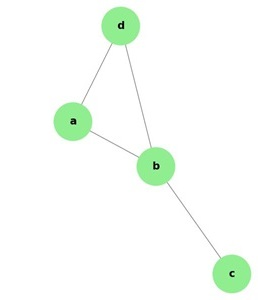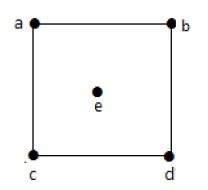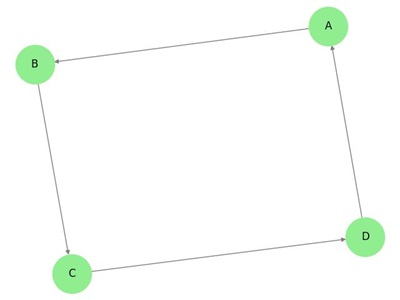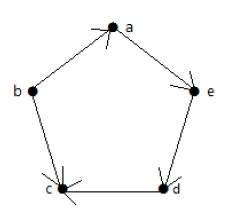# Degree of Vertex of a Graph

It is the number of vertices adjacent to a vertex V.

Notation − deg(V).

In a simple graph with n number of vertices, the degree of any vertices is −

deg(v) = n – 1 ∀ v ∈ G

A vertex can form an edge with all other vertices except by itself. So the degree of a vertex will be up to the number of vertices in the graph minus 1. This 1 is for the self-vertex as it cannot form a loop by itself. If there is a loop at any of the vertices, then it is not a Simple Graph.

Degree of vertex can be considered under two cases of graphs −

• Undirected Graph
• Directed Graph

## Degree of Vertex in an Undirected Graph

An undirected graph has no directed edges. Consider the following examples.

## Example 1

Take a look at the following graph −In the above Undirected Graph,

• deg(a) = 2, as there are 2 edges meeting at vertex 'a'.

• deg(b) = 3, as there are 3 edges meeting at vertex 'b'.

• deg(c) = 1, as there is 1 edge formed at vertex 'c'

So 'c' is a pendent vertex.

• deg(d) = 2, as there are 2 edges meeting at vertex 'd'.

• deg(e) = 0, as there are 0 edges formed at vertex 'e'.

So 'e' is an isolated vertex.

## Example 2

Take a look at the following graph −In the above graph,

deg(a) = 2, deg(b) = 2, deg(c) = 2, deg(d) = 2, and deg(e) = 0.

The vertex 'e' is an isolated vertex. The graph does not have any pendent vertex.

## Degree of Vertex in a Directed Graph

In a directed graph, each vertex has an indegree and an outdegree.

## Indegree of a Graph

• Indegree of vertex V is the number of edges which are coming into the vertex V.

• Notation − deg(V).

## Outdegree of a Graph

• Outdegree of vertex V is the number of edges which are going out from the vertex V.

• Notation − deg+(V).

Consider the following examples.

## Example 1

Take a look at the following directed graph. Vertex 'a' has two edges, 'ad' and 'ab', which are going outwards. Hence its outdegree is 2. Similarly, there is an edge 'ga', coming towards vertex 'a'. Hence the indegree of 'a' is 1.The indegree and outdegree of other vertices are shown in the following table −

VertexIndegreeOutdegree
a12
b20
c21
d11
e11
f11
g02

## Example 2

Take a look at the following directed graph. Vertex 'a' has an edge 'ae' going outwards from vertex 'a'. Hence its outdegree is 1. Similarly, the graph has an edge 'ba' coming towards vertex 'a'. Hence the indegree of 'a' is 1.The indegree and outdegree of other vertices are shown in the following table −

VertexIndegreeOutdegree
a11
b02
c20
d11
e11